Math resources Geometry 2D shapes

How to draw a hexagon

# How to draw a hexagon

Here you will learn about how to draw a hexagon including how to recognize and draw a hexagon using different techniques.

Students will first learn about how to draw a hexagon as part of geometry in 2 nd grade.

## What is a hexagon and how do you draw a hexagon?

How to draw a hexagon is creating a six-sided figure with six vertices by drawing simple lines and shapes and connecting them to one another. To do this, you will need to use a pencil and a ruler (a straight-edge).

For example, you can draw a hexagon within a circle.

A hexagon is a closed two-dimensional polygon made up of six straight sides. A regular hexagon is a regular polygon with six sides and six vertices. It has equal sides and equal angles.

An irregular hexagon is a six-sided shape, where its six sides are not equal in length to each other.

For example,

Other geometric shapes can be constructed in a similar way. Equilateral triangles, squares and regular hexagons along with other regular polygons including regular pentagons, regular heptagons and regular octagons can be easily drawn and have been used in art for many centuries.

### What is a hexagon and how do you draw a hexagon?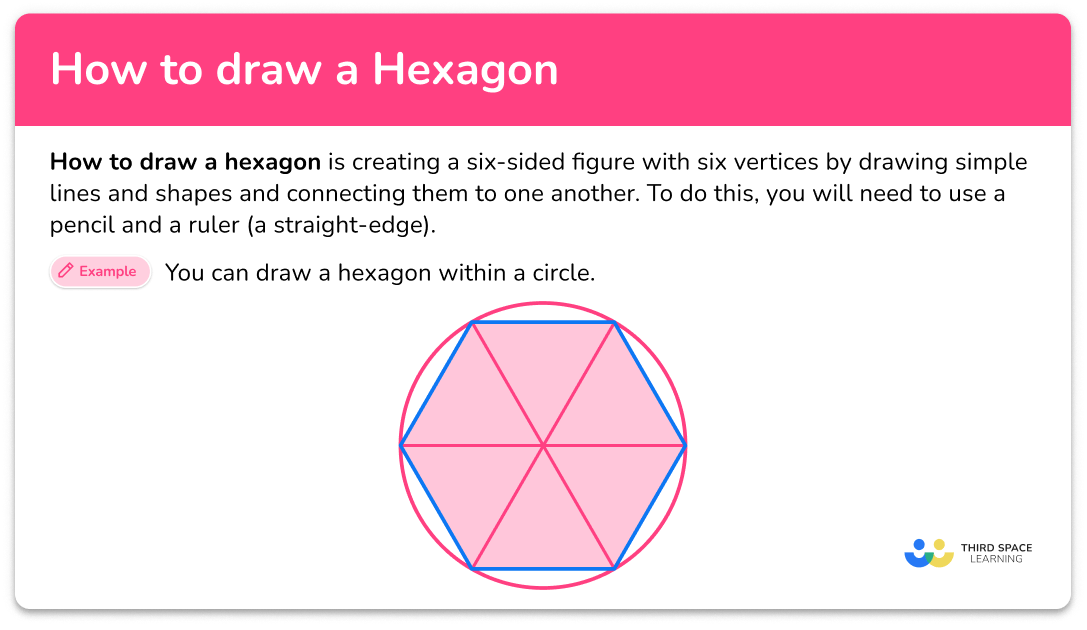## Common Core State Standards

How does this relate to 2 nd grade math?

Recognize and draw shapes having specified attributes, such as a given number of angles or a given number of equal faces. Identify triangles, quadrilaterals, pentagons, hexagons, and cubes.

## How to draw a regular hexagon

In order to draw a regular hexagon:

1. Draw a horizontal line as the base of the hexagon.
2. From each end of the line segment, draw a short vertical line that extends upwards.
3. Drawing another vertical line that connects to the end of each line segment.
4. Connect the final two line segments with another horizontal line as the top of the shape.
5. Check that your shape appears to have sides of equal length.

## How to draw a hexagon examples

### Example 1: draw a hexagon

Construct a hexagon.

1. Draw a horizontal line as the base of the hexagon.

2From each end of the line segment, draw a short vertical line that extends upwards.

3Drawing another vertical line that connects to the end of each line segment.

4Connect the final two line segments with another horizontal line as the top of the shape.

5Check that your shape appears to have sides of equal length.

Check that the sides appear to be equal length and that there is the correct number of sides.

## How to draw a regular hexagon in a circle

In order to draw a regular hexagon in a circle:

1. Draw a large circle and mark the center of the circle.
2. Draw a horizontal line through the center of the circle.
3. Draw two diagonal lines (an x) through the center of the circle.
4. Connect the points where the lines intersect the circle.
5. Erase the circle and original lines drawn (horizontal and diagonal lines).

### Example 2: draw a regular hexagon in a circle

Construct a regular hexagon within a circle.

The use of a ruler or straight edge will help make sure the line is straight.

When drawing the two diagonal lines, take a moment to review the drawing to ensure that the pieces of the circle are approximately the same size.

Note: To draw an irregular hexagon, the circle could be split into different sized pieces, instead of equal pieces.

The use of a ruler or straight edge will help make sure the lines are straight. Review the drawing to make sure that the lines are approximately the same length.

Check that the sides appear to be equal length and that there is the correct number of sides.

## How to draw a hexagon from a square

In order to draw a hexagon from a square:

1. Draw a square.
2. Locate the midpoint of one side of the square and mark with a dot. Repeat with the opposite side of the square.
3. Split the other opposite sides into three.
4. Cut the corners off the square using diagonal lines.
5. Erase any unneeded lines and marks, leaving only the hexagon.

### Example 3: draw a hexagon from a square

The use of a ruler or straight edge will help make sure the lines are straight.

The use of a ruler or straight edge will help make sure the lines are straight.

### Teaching tips for how to draw a hexagon

• For students first learning how to draw hexagons or beginners, you can provide worksheets that have trace lines with simple steps for students to practice with. Students can then graduate to using a template of a circle or square to practice. As students become more comfortable with drawing the shape, they can try freehand.

• Students can benefit from having a video to watch the different ways to draw a hexagon repeatedly. Creating or finding a step-by-step drawing tutorial online is one way for students to have access to this.

### Easy mistakes to make

• Not using exact measurements when drawing a regular hexagon
When drawing a regular hexagon, the sides of the hexagon must be equal length. While an advanced artist may be able to do this freehand, while learning and perfecting your form, the use of a ruler and other tools will ensure that you are getting exact measurements.

### Practice how to draw a hexagon questions

1) Which drawing below appears to be a regular hexagon?A hexagon is a closed, 2D shape made up of six straight sides and six vertices.

The shapes in these drawings do not have 6 sides,This drawing shows a regular hexagon,2) Which drawing below appears to be a regular hexagon?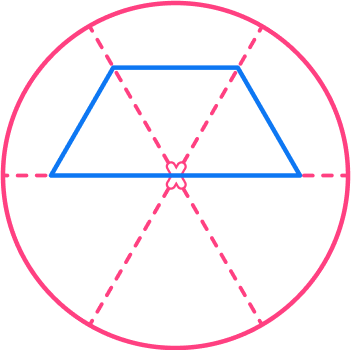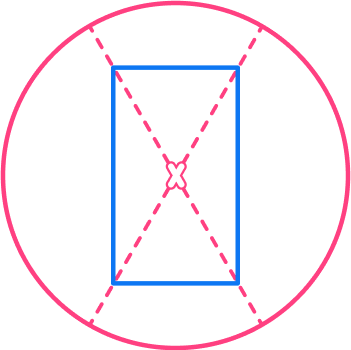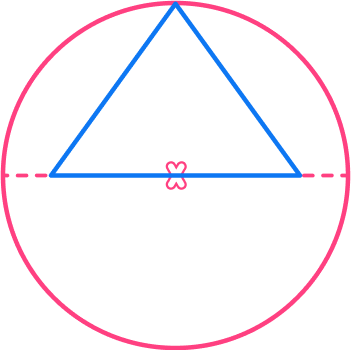A hexagon is a closed, 2D shape made up of six straight sides and six vertices.

The shapes in these drawings do not have 6 sides,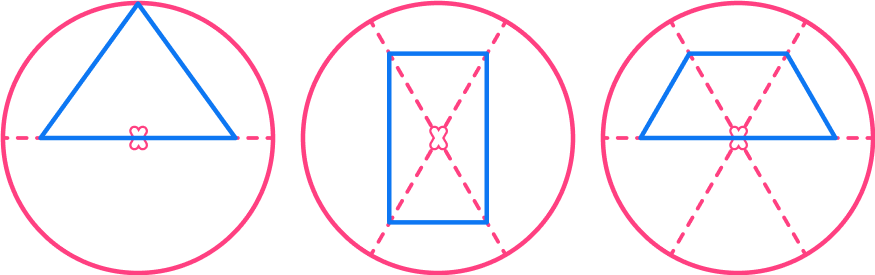This drawing shows a regular hexagon,3) Which drawing below appears to show a hexagon in a square?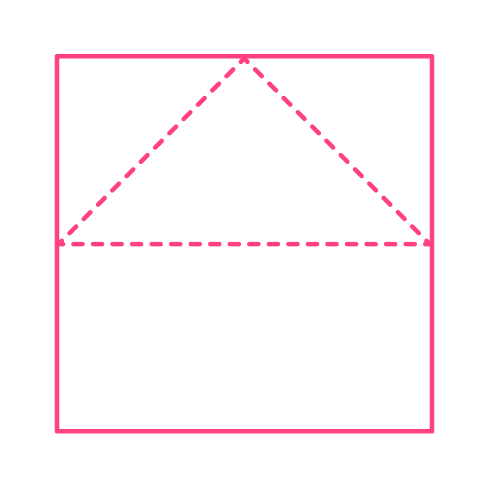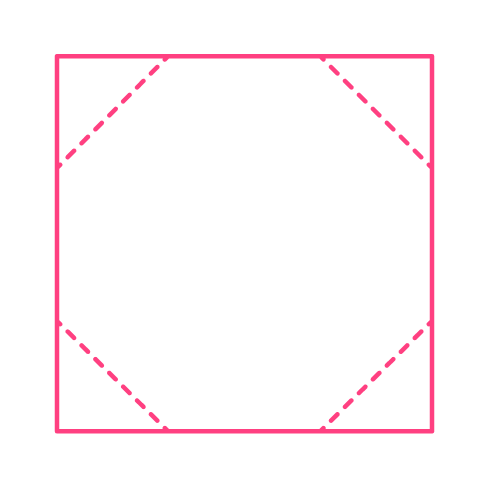A hexagon is a closed, 2D shape made up of six straight sides and six vertices.

The shapes in these drawings do not have 6 sides,This drawing shows a hexagon in a square.## How to draw a hexagon FAQs

Can I draw a hexagon with or without any tools?

While using a ruler can help make straight lines and equal sides, it’s not necessary for drawing a hexagon. You can still draw a hexagon freehand, but using a ruler will help maintain exact measurements.

How can I make my hexagon symmetrical and even?

To make your hexagon symmetrical and even, pay attention to the angles and lengths of each side. Take your time when drawing and use measuring tools like a ruler or protractor to ensure accuracy. Practice and patience will help you improve your skills and create more perfect hexagons.

What does the name “hexagon” mean?

The name hexagon is divided into ‘hex’ meaning six, and ‘gonia’ meaning apex or corners.

## Still stuck?

At Third Space Learning, we specialize in helping teachers and school leaders to provide personalized math support for more of their students through high-quality, online one-on-one math tutoring delivered by subject experts.

Each week, our tutors support thousands of students who are at risk of not meeting their grade-level expectations, and help accelerate their progress and boost their confidence.